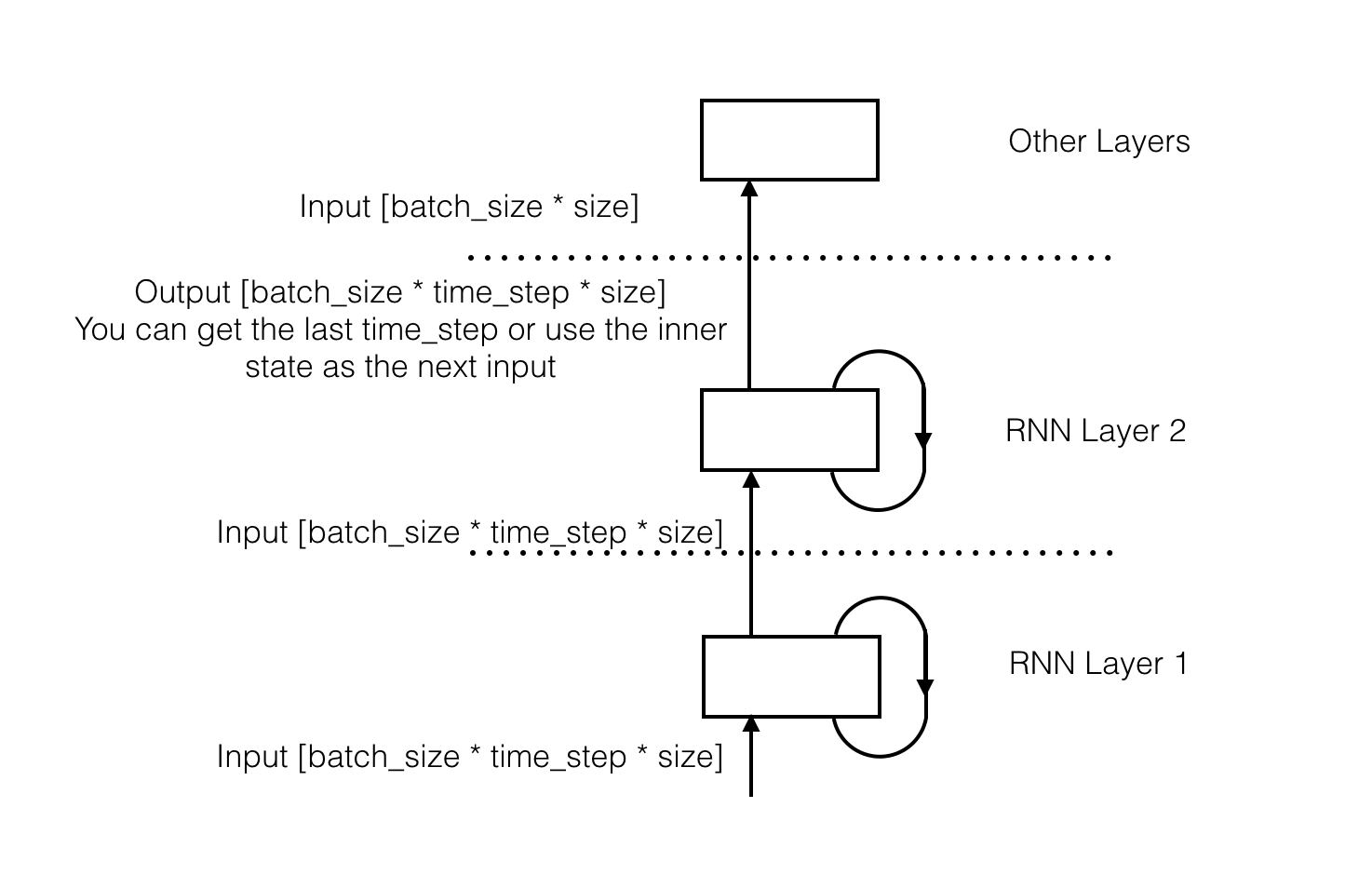# How to Put RNN Layers Into Neural Network Model

Posted on 14 May 2016, tagged neural nerworkrnn

If you are interested in neural networks, you must have ever read and learn lots of things about RNN, which can reason about sequences of data. RNN is awesome and very fun to play with, if you haven’t heard of it, you can read this great article which train a char level RNN model to generate text.

While these articles are very great and introduce the idea and structure of RNN, but they didn’t mention the details of how to put an RNN layer into your neural network model. I’ve reimplemented char level RNN these days two times, one with Theano and the other one with TensorFlow. I’ll talk about how to fit a RNN layer into a neural network model.

## Basic Idea of RNN

The basic idea of RNN is that you have a sequence of data, you can train on this data so that represent the sequence of data as a vector, then you can do other tricks on this trained vector in other tasks. So make it simple: RNN deals with sequence data (maybe dymical length).

For example, given this task: the input is a sequence of chars, and you need to predict the next char of the sequence. Then you can train to represent the sequence of chars as a vector with RNN, and then input the vector into next layer, maybe a linear layer with softmax activation, like in other classification tasks.

So how does RNN do this? RNN will do these things:

• Step over the sequence of data.

For each step:

• Count next state based on the current step data and current state: $$next\_state = state\_func(state, input_i)$$
• Count output from current input and state: $$output_i = out\_func(state, input_i)$$
• Update the state: $$state = next\_state$$

The state_func and out_func maybe different depends on what kind of the RNN is (for example, LSTM or GRU), but the basic structure is the same.

A thing we need to notice is, the process I just described, is just the behaviour of one RNN layer.

Then we’ve got two outputs from the RNN: a sequence of output and its inner state. Normally, if we want to stack multiple RNN layers, we can use the sequence of output as the next input of RNN layer. If you just want a vector to represent the sequence of data, you can get the output of the last step. Some other models such as seq2seq also uses the inner state to represent the sequence of data.

## Feed the Input

We have the basic idea of RNN. How do we implement it efficiently? If we want our model run fast, we need to run it on GPU. So in order to make it fast, we should:

• Copy batch of inputs into the GPU instead of run them one by one. So use mini-batch training.
• Represent the data as tensor and use tensor operations as many as you could. (Many BLAS library and deep learning frameworks will do a lot of optimizations on tensor operations).

Based on this, we should represent the input data as tensor. We may need these dimensions for the tensor:

• Batch size to represent multiple batches.
• Time step to represent the sequence of data.
• Vector size for each item of the input sequence. (If each item of the input sequence cannot be represent as a vector, you may need other dimensions to represent the item. )

So the shape of the tensor is [batch_size * time_step * size]. With this regular tensor, we can fit our RNN layer into the neural network model.## Some Other Details

### Loop Operations

Since we want to use tensor operations as many as we could, we don’t want to split the tensor based on the time_step dimension and use our own loop. Some deep learning frameworks have there own loop operations. These operations should be optimized to be more efficient.

Theana and TensorFlow both use symbol computation. They both have the loop operation, too. It’s scan in Theano and while_loop in TensorFlow.

### Variable Time Step

Some input sequences of RNN have different length. One way to solve this is use the max length as time step, and padding other samples with some special value like zero. But this can be expensive. Another way is split the sequences into many tensors with the same time step, and let RNN keep its inner state while training on a same sequence. The implement can be tricky, you can see the implement of Keras.

 Prev: The Permission Management of Android Becomes A Bigger Problem When It Comes to Wearable Devices and TV Next: Build A Computer for Deep Learning